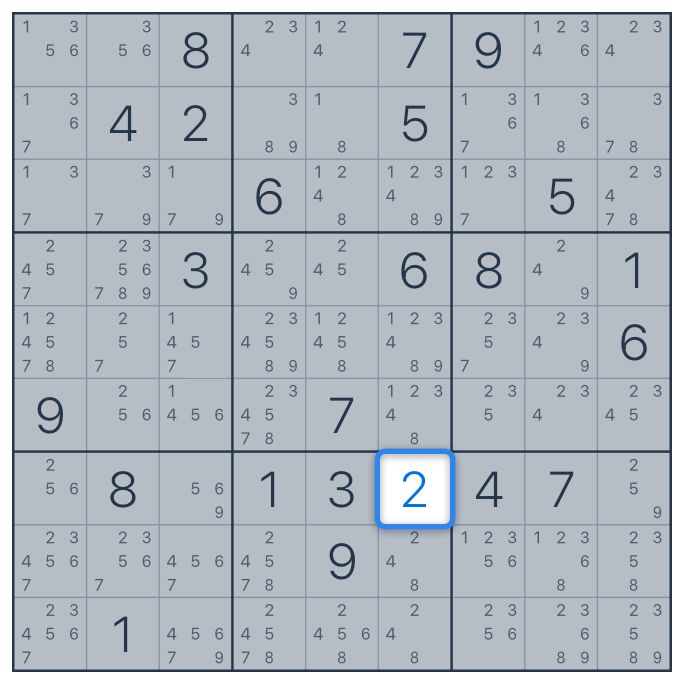# "Obvious singles" technique.

This strategy is based on the correct placement of Notes. Sometimes it is called Naked Singles. The point is that in a specific cell only one digit (from the Notes) remains possible.

Let's have look at this case with an example.Let's look at the highlighted cell. We can see that it is filled with only one Note - number 2. It means that this cell has only one possible solution. Since it is the only possible option, this cell will be 2. So we remove Note from this cell and fill it with the number 2.

That is how "Obvious singles" technique works. As you see, this is not as difficult as it seems. Therefore, if you put "Obvious singles" technique into practice, the process of solving Sudoku will become easier and faster!